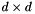So-Bogus A c++ sparse block matrix library aimed at Second Order cone problems
bogus::DualFrictionProblem< Dimension > Struct Template Reference

Dual representation of a Coulomb friction problem, with explicit Delassus operator. More...

#include <FrictionProblem.hpp>

## Public Types

typedef SparseBlockMatrix
< Eigen::Matrix< double,
Dimension, Dimension,
Eigen::RowMajor >, SYMMETRIC >
WType

typedef GaussSeidel< WTypeGaussSeidelType

typedef ProjectedGradient< WTypeProjectedGradientType

typedef SOCLaw< Dimension,
double, true >
CoulombLawType

typedef SOCLaw< Dimension,
double, false >
SOCLawType

typedef Signal< unsigned, double > SignalType

## Public Member Functions

void computeFrom (const PrimalFrictionProblem< Dimension > &primal)
Computes this DualFrictionProblem from the given primal. More...

double solveWith (GaussSeidelType &gs, double *r, const bool staticProblem=false) const
Solves this problem. More...

double solveWith (ProjectedGradientType &pg, double *r) const

double evalWith (const GaussSeidelType &gs, const double *r, const bool staticProblem=false) const
Evaluate a residual using the GS's error function. More...

double evalWith (const ProjectedGradientType &gs, const double *r) const

double solveCadoux (GaussSeidelType &gs, double *r, const unsigned fpIterations, const SignalType *callback=(static_cast< const SignalType * >(0))) const
Solves this problem using the Cadoux algorithm ( with fixed-point iteration ) More...

double solveCadoux (ProjectedGradientType &pg, double *r, const unsigned fpIterations, const SignalType *callback=(static_cast< const SignalType * >(0))) const

double solveCadouxVel (GaussSeidelType &gs, double *u, const unsigned fpIterations, const SignalType *callback=(static_cast< const SignalType * >(0))) const
Idem as solveCadoux, but interpreting the problem as r = Wu + b.

double solveCadouxVel (ProjectedGradientType &pg, double *u, const unsigned fpIterations, const SignalType *callback=(static_cast< const SignalType * >(0))) const

void applyPermutation (const std::vector< std::size_t > &permutation)
Apply a permutation on the contact indices. More...

void undoPermutation ()

bool permuted () const

const std::vector< std::size_t > & permutation () const

const std::vector< std::size_t > & invPermutation () const

## Public Attributes

WType W
W – Delassus operator.

Eigen::VectorXd b
Rhs ( such that u = Wr + b )

Eigen::VectorXd mu
Coulomb friction coefficients.

## Detailed Description

### template<unsigned Dimension> struct bogus::DualFrictionProblem< Dimension >

Dual representation of a Coulomb friction problem, with explicit Delassus operator.

u = W r + b such that u and r satisfy CoulombLaw(mu) or SOCLaw(mu), where W is a symmetric, positive semi-definite matrix withblocks, u are the relative velocities and r are the contact forces. May be constructed from a PrimalFrictionProblem, or by directly initializing each data member to accomodate problems with different mass matrix structures. See also Constrained Iterative Solvers .

## Member Function Documentation

template<unsigned Dimension>
 void bogus::DualFrictionProblem< Dimension >::applyPermutation ( const std::vector< std::size_t > & permutation )

Apply a permutation on the contact indices.

Useful for achieving better memory locality when using the Coloring functionality of the GaussSeidel algorithm.

See Also
Coloring
Warning
To use the permutation releated functions, all the blocks have to have the same size
template<unsigned Dimension>
 void bogus::DualFrictionProblem< Dimension >::computeFrom ( const PrimalFrictionProblem< Dimension > & primal )

Computes this DualFrictionProblem from the given primal.

Warning
Assumes MInv has been computed
template<unsigned Dimension>
 double bogus::DualFrictionProblem< Dimension >::evalWith ( const GaussSeidelType & gs, const double * r, const bool staticProblem = false ) const

Evaluate a residual using the GS's error function.

Parameters
 gs The GaussSeidel< WType > solver to use r Both the current force staticProblem If true, eval this problem as a SOCQP instead of a Coulomb Friction problem
Returns
the error as returned by the GaussSeidel::eval() function
template<unsigned Dimension>
 double bogus::DualFrictionProblem< Dimension >::solveCadoux ( GaussSeidelType & gs, double * r, const unsigned fpIterations, const SignalType * callback = (static_cast< const SignalType *>(0)) ) const

Solves this problem using the Cadoux algorithm ( with fixed-point iteration )

See 

Parameters
 gs The GaussSeidel< WType > solver to use r Both the initial guess and the result fpIterations Number of fixed-point iterations callback 0, or a pointer to a user-defined function that takes ( unsigned iteration, double residual ) as arguments
Returns
the error as returned by the GaussSeidel::solve() function
template<unsigned Dimension>
 double bogus::DualFrictionProblem< Dimension >::solveWith ( GaussSeidelType & gs, double * r, const bool staticProblem = false ) const

Solves this problem.

Parameters
 gs The GaussSeidel< WType > solver to use r Both the initial guess and the result staticProblem If true, solve this problem as a SOCQP instead of a Coulomb Friction problem
Returns
the error as returned by the GaussSeidel::solve() function

The documentation for this struct was generated from the following files: